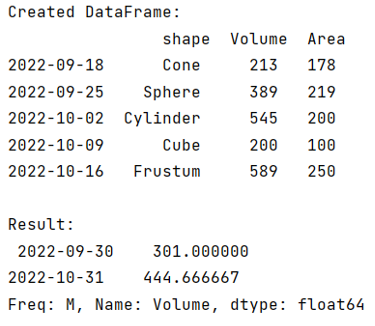# Pandas DataFrame Resample

Learn about the Pandas dataframe resample, its concept with examples.
Submitted by Pranit Sharma, on September 17, 2022

Pandas is a special tool that allows us to perform complex manipulations of data effectively and efficiently. Inside pandas, we mostly deal with a dataset in the form of DataFrame. DataFrames are 2-dimensional data structures in pandas. DataFrames consist of rows, columns, and data.

## DataFrame Resample

Pandas DataFrame.resample() method is used for time series data. A time series is a series of data points that are listed or ranked in time order. Most commonly, a time series can also be considered as a sequence defined at continuous equally spaced points in time.

It is an appropriate method for frequency conversion and resampling of time series.

Syntax:

```DataFrame.resample(
rule,
axis=0,
closed=None,
label=None,
convention='start',
kind=None,
loffset=None,
base=None,
on=None,
level=None,
origin='start_day',
offset=None
)
```

Parameters:

• rule: Target conversion
• axis: Column-wise or row-wise
• closed: Right or left boundary
• label: Right or left label
• convention: For PeriodIndex only, controls whether to use the start or end of rule.
• kind: Pass 'timestamp' to convert the resulting index to a DateTimeIndex or 'period' to convert it to a PeriodIndex. By default the input representation is retained.
• base: For frequencies that evenly subdivide 1 day, the "origin" of the aggregated intervals.
• on: column to use in case not the index is passed
• level: usually used for multiindex DataFrames

Let us understand with the help of an example,

## Python code for to demonstrate example of Pandas DataFrame Resample

```# Importing pandas package
import pandas as pd

# Creating dictionary
d = {
'shape':['Cone','Sphere','Cylinder','Cube','Frustum'],
'Volume':[213,389,545,200,589],
'Area':[178,219,200,100,250]
}

# Creating DataFrame
df = pd.DataFrame(d,index=pd.date_range('09/16/2022',
periods=5,freq='W'))

# Display Original DataFrames
print("Created DataFrame:\n",df,"\n")

# Using resample method
res = df.Volume.resample('M').mean()

# Display Result
print("Result:\n",res)
```

Output:Reference:

What's New (MCQs)

Top Interview Coding Problems/Challenges!

Languages: » C » C++ » C++ STL » Java » Data Structure » C#.Net » Android » Kotlin » SQL
Web Technologies: » PHP » Python » JavaScript » CSS » Ajax » Node.js » Web programming/HTML
Solved programs: » C » C++ » DS » Java » C#
Aptitude que. & ans.: » C » C++ » Java » DBMS
Interview que. & ans.: » C » Embedded C » Java » SEO » HR
CS Subjects: » CS Basics » O.S. » Networks » DBMS » Embedded Systems » Cloud Computing
» Machine learning » CS Organizations » Linux » DOS
More: » Articles » Puzzles » News/Updates

© https://www.includehelp.com some rights reserved.